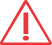You are here: Home > Practicals > Measuring the Speed of Light
Practicals
Categories
Physics
- EM Waves
Options

# Measuring the Speed of Light AKA: Microwaves and Chocolate

## Summary

A chocolate bar is heated briefly in a microwave oven and the speed of light calculated from the resultant distance between melted areas.

## Operation

This is a famous demonstration involving a microwave oven and a chocolate bar. Any large chocolate bar may be used for this - the large 'A5' sized bars are perfect. The microwave also needs a bit of modification- the revolving plate needs to be removed first in order for it to work.

The chocolate bar is placed inside the microwave oven with the revolving plate absent. The plate usually makes sure that every part of the food being heated is exposed to the beam of microwaves. With the plate removed it means that the microwaves are not being spread evenly throughout the oven and so 'hot spots' occur which melt specific areas of the chocolate surface. Sufficient melting usually occurs after only 30-40 seconds.

The distance between the hot spots represents half the wavelength of the microwaves being emitted from the oven. Let's say that you measure this distance at 6cm and double it to calculate a wavelength of 12cm. This figure needs to be multiplied by the frequency of the microwave. This frequency of most microwave ovens is 2.45GHz, that is, 2,450,000,000 Hz. The exact frequency is printed on the back of the device. Multiplying the two calculates the speed of the wave (which is the speed of light) in cm/s.

12 x 2,450,000,000 = 29,400,000,000

The resultant figure should be divided by 100 to convert it to m/s. The speed of light is 299,782,458. The exact figure would be near-impossible to reach using the chocolate method but you can get very close.

We use the following equation to find out the speed of light:

λ=
 v f

Where λ = wavelength, v = speed and f = frequency.

The microwave oven should be used only for scientific experimentation such as this demonstration as powering the oven without the rotating plate may damage the device.

## SafetyThe contents of this page are for information only. Please refer to CLEAPSS, SSERC or ASE safety advice and/or publications before undertaking any preparation, practical experiment or using any equipment featured on this site or any other.